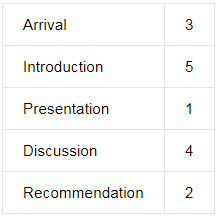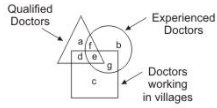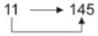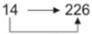Courses

# JIPMER Aptitude Mock Test 1

## 10 Questions MCQ Test JIPMER 2020: Subject wise and Full Length Mock Tests | JIPMER Aptitude Mock Test 1

Description
This mock test of JIPMER Aptitude Mock Test 1 for NEET helps you for every NEET entrance exam. This contains 10 Multiple Choice Questions for NEET JIPMER Aptitude Mock Test 1 (mcq) to study with solutions a complete question bank. The solved questions answers in this JIPMER Aptitude Mock Test 1 quiz give you a good mix of easy questions and tough questions. NEET students definitely take this JIPMER Aptitude Mock Test 1 exercise for a better result in the exam. You can find other JIPMER Aptitude Mock Test 1 extra questions, long questions & short questions for NEET on EduRev as well by searching above.
QUESTION: 1

Solution:

A
E
C
F
B
D

QUESTION: 2

### Arrange the words given below in a meaningful sequence. 1. Presentation 2. Recommendation 3. Arrival 4. Discussion 5. Introduction

Solution:

The correct order is :QUESTION: 3

### If + means -, - means x, x means ÷ and ÷ means +, then 36 x 2 + 8 ÷ 8 = ?

Solution:
QUESTION: 4

Which is the Number that comes next in the series:   2, 1, (1 / 2), (1 / 4), .?

Solution:

This is a simple division series; each number is one-half of the previous number.

QUESTION: 5

Below is three statements followed by four conclusions numbered I,II,III and IV. You have to consider the statement and the following conclusions and decide which of the conclusions is follows in the statement :
Statement:
a.Some cruel animals are papers.
b. No paper is tree.
c. All trees are ways.
Conclusions: I. No cruel animal is tree.
II. Some ways are trees.
III. Some papers are cruel animals.
IV. Some cruel animals are trees.

Solution:
QUESTION: 6

If all those numbers from 1-40 each of which is exactly divisible by 4 and/or has 4 as a digit in it are removed, how many numbers will remain?

Solution:

Numbers exactly divisible by 4 are table of 4 and 14 are 34 have 4 as a digit so the numbers which left are 40 -12 = 28.

QUESTION: 7

Three persons A, B and C are standing in queue. There are five persons between A and B and eight persons between B and C. If there ne three persons ahead of C and 21 persons behind A, what could be the minimum number of persons in the queue ?

Solution:

Three persons A, B, C can be arranged in a queue in six different ways
i.e., ABC, CBA, BAC, CAB, BCA, ACB
But since there are only 3 persons ahead of C,
So, C should be in front of the queue
Thus, there are only two possible arrangements
i.e., CBA and CAB. We may consider the two cases as under :
clearly, number of persons in the queue = (3 + 1 + 8 + 1 + 5 + 1 + 21) = 40
Number of persons between A and C = (8 - 6) = 2
Clearly, number of persons in the queue = (3 + 1 + 2 + 1 + 21) = 28.
Now, 28 < 40
So, 28 is the minimum number of perosn in the queue.

QUESTION: 8

With the help of the given diagram, answer the following questions making the right choice from the given alternatives. Indicate the answer as per the "Instructions"Q. Qualified and experienced doctors working in villages are represented by the letter .....

Solution:

Square represents doctors in rural areas
Triangle represents qualified doctors
Circle represents experienced doctors
All shapes(units) will meet at area "e" So answer is "e"

QUESTION: 9

Complete the analogous pair.
11 : 145 :: 14 : ?

Solution:(11 + 1)2 + 1(14 + 1)2 + 1

QUESTION: 10

Each question consists of four sets of three statements each. Identify the set in which the third statement follows logically from the first two.

P. Some fruits are sour. Some apples are sour. All sour are either fruits or apples.
Q. Some As are Bs. All Cs are As. Most Cs are Bs.
R. All birds lay eggs. An ostrich is a bird. An ostrich lays eggs.
S. All As need B. Cs are As. Cs need B.

Solution:

All birds lay eggs + An ostrich is a bird = An ostirch lays eggs.

And All As need B + Cs are As = Cs need B.